## Time complexity of HAC

The complexity of the naive HAC algorithm in Figure 17.2 is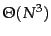because we exhaustively scan the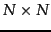matrix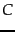for the largest similarity in each of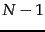iterations.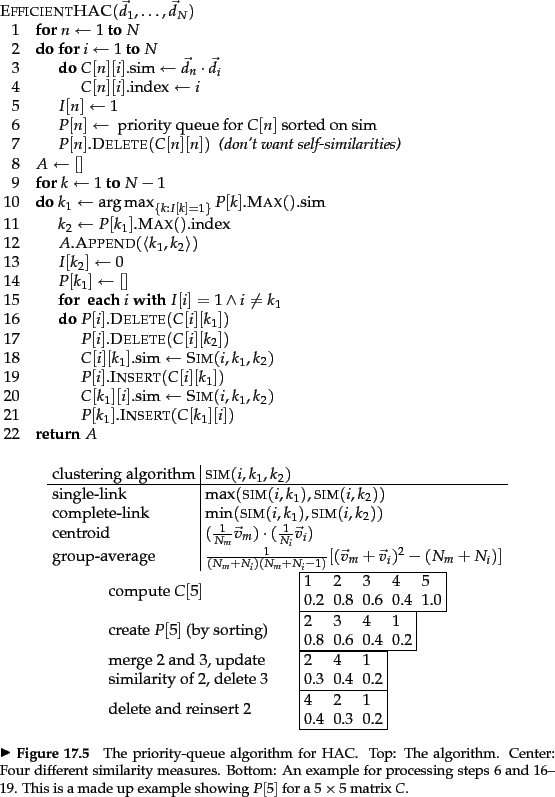For the four HAC methods discussed in this chapter a more efficient algorithm is the priority-queue algorithm shown in Figure 17.8 . Its time complexity is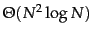. The rows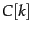of thesimilarity matrixare sorted in decreasing order of similarity in the priority queues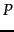.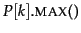then returns the cluster in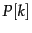that currently has the highest similarity with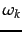, where we useto denote the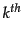cluster as in Chapter 16 . After creating the merged cluster of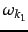and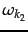,is used as its representative. The function SIM computes the similarity function for potential merge pairs: largest similarity for single-link, smallest similarity for complete-link, average similarity for GAAC (Section 17.3 ), and centroid similarity for centroid clustering (Section 17.4 ). We give an example of how a row ofis processed (Figure 17.8 , bottom panel). The loop in lines 1-7 is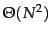and the loop in lines 9-21 isfor an implementation of priority queues that supports deletion and insertion in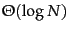. The overall complexity of the algorithm is therefore. In the definition of the function SIM,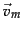and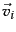are the vector sums of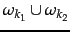and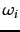, respectively, and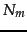and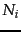are the number of documents inand, respectively.

The argument of EFFICIENTHAC in Figure 17.8 is a set of vectors (as opposed to a set of generic documents) because GAAC and centroid clustering ( and 17.4 ) require vectors as input. The complete-link version of EFFICIENTHAC can also be applied to documents that are not represented as vectors.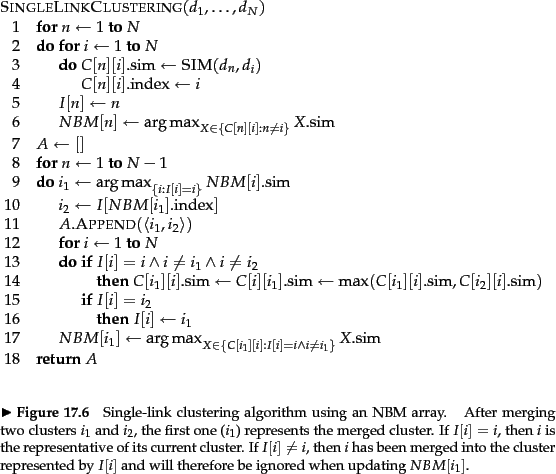For single-link, we can introduce a next-best-merge array (NBM) as a further optimization as shown in Figure 17.9 . NBM keeps track of what the best merge is for each cluster. Each of the two top level for-loops in Figure 17.9 are, thus the overall complexity of single-link clustering is.

Can we also speed up the other three HAC algorithms with an NBM array? We cannot because only single-link clustering is best-merge persistent . Suppose that the best merge cluster foris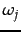in single-link clustering. Then after mergingwith a third cluster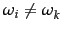, the merge ofandwill be's best merge cluster (Exercise 17.10 ). In other words, the best-merge candidate for the merged cluster is one of the two best-merge candidates of its components in single-link clustering. This means thatcan be updated in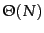in each iteration - by taking a simple max of two values on line 14 in Figure 17.9 for each of the remaining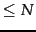clusters.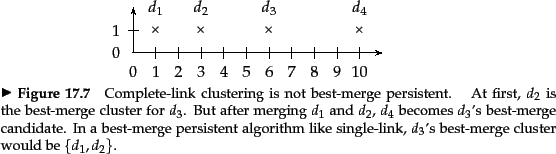Figure 17.10 demonstrates that best-merge persistence does not hold for complete-link clustering, which means that we cannot use an NBM array to speed up clustering. After merging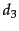's best merge candidate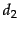with cluster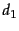, an unrelated cluster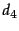becomes the best merge candidate for. This is because the complete-link merge criterion is non-local and can be affected by points at a great distance from the area where two merge candidates meet.

In practice, the efficiency penalty of thealgorithm is small compared with thesingle-link algorithm since computing the similarity between two documents (e.g., as a dot product) is an order of magnitude slower than comparing two scalars in sorting. All four HAC algorithms in this chapter arewith respect to similarity computations. So the difference in complexity is rarely a concern in practice when choosing one of the algorithms.

Exercises.

• Show that complete-link clustering creates the two-cluster clustering depicted in Figure 17.7 .